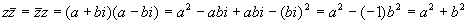The portion of the formula under the radical is called the discriminant, and determines which of the three cases is under consideration.

In this case we haveSo there are two different roots, both real numbers.

Example

Find the roots (solve for x):Solution

Plug the numbers into the quadratic formulaAs a check, plug these solutions into a generic (parentheses or FOIL) formMultiplying the last expression by 2 yields the above quadratic.

Example

Find the roots (solve for x):SolutionThus the two roots areDo a FOIL check to make sure these are correctThis is a good calculation to go through carefully, since it contains lots of opportunities for errors. Sticking to the FOIL method helps, as does proper use of parentheses and brackets. Instructors love putting these types of problem on exams, since they really test the students' organizational skills and attention to detail.

This is the case where the discriminant is zero…and so the expression under the radical vanishes, leaving nothing after the +/-. This corresponds to the graph having only the vertex touching the x-axis, so there is exactly one real root.

Example

Find the roots (solve for x):SolutionNow do a check:…upon division by 2. Note that it is very difficult to see that this equation is a perfect square by inspection.

Example

Find the roots (solve for x)SolutionAs a check, multiply out the square:A few short comments about complex numbers are appropriate here. As we saw in the Quadratic Theory section, some quadratics do not cross the x-axis at all. For example, the quadraticnever gets closer than 1 to the x-axis, and so has no real zeros at all. However, if we try to solve it anyway,The symbol 'i' is an abbreviation for the square root of minus one, and is called the imaginary unit. Any number that includes this symbol is a complex number (not a real number). For the time being, the only thing we care about i is that i2 = -1. A general complex number is usually writtenwhere a and b are real numbers (the real part and imaginary part respectively). Every complex number has a complex conjugatewhere the sign of the imaginary part is reversed. Complex conjugate pairs of numbers are interesting because their products are always real:In fact, if either a or b is non-zero, this product is positive.

Example

Solve for xSolutionPlug into the FOIL to verifyNote that we used the results from the product of complex conjugates to simplify the product of the roots.

Example

Solve for xSolutionAnd then do a FOIL to checkThe classic algebra problem book - very light on theory, plenty of problems with full solutions, more problems with answers

A simplified and updated version of the classic Schaum's Outline.   Not as complete as the previous book, but enough for most students